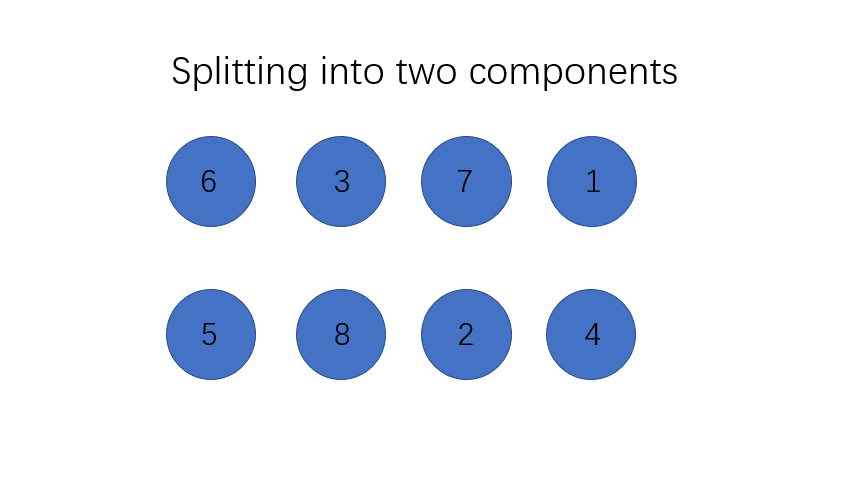# Count of Smaller Numbers after Self | Number of Swaps to Sort | Algorithm Swap

You are given an integer array nums and you have to return a new counts array. The counts array has the property where `counts[i]` is the number of smaller elements to the right of `nums[i]`.

Input:

``1    [5,2,6,1]``

Output:

``1    [2,1,1,0]``

Explanation:

For the number 5, there are 2 numbers smaller than it after it. (2 and 1)

For the number 2, there is 1 number smaller than it after it. (1)

For the number 6, there is also 1 number smaller than it after it. (1)

For the number 1, there are no numbers smaller than it after it.

Hence, we have `[2, 1, 1, 0]`.

## Number of swaps to sort

Another way to phrase the question is:

If we sort the array by finding the smallest pair `i, j` where `i < j` and `a[i] > a[j]` how many swaps are needed?

To answer that question we just have to sum up the numbers in the above output array: `2 + 1 + 1 = 4` swaps.

### Explanation

#### Intuition

The brute force way to solve this question is really easy and intuitive, we simply go through the list of elements. For each of the element, we go through the elements after it and count how many numbers are smaller than it. This would result in a O(N^2) runtime. However, this approach is not the optimal solution.

Observe that if we need to reduce our solution's complexity, we will need to count multiple numbers' smaller count in one go. This can only be done using some kind of sorted order.

But sorting destroys the origin order of the array, what can we do about that?

Recall from introduction of divide and conquer questions, the common approach of tackling a divide and conquer question is dividing the data given into two components, assuming each components is solved and then try to merge the result.

What if we divide the numbers into two components by index and then sort them separate?

Since we divided the original array by index, after the two components are both sorted, all the elements in the left components still have smaller index than any element in the right components in the original array.

We can utilize this fact when we combine the two arrays together.

Thus, to solve this problem, we first split the data given into two components, the left and the right components. And then we assume that both components' sub-problem are already solved -- that is we know the count of number smaller than itself for each number for both components. Now all we need to know is for each number in the left component, how many elements are smaller than it in the right component.This will allow us to know for each number in the left components, how many elements is smaller than it in the right component.

Thus, we have successfully solved the problem.

So, what is the run time of our improved solution? We split the problem into two components each recursion and go through each of the components, and each recursion takes O(N) time for the merge process. Thus we have

``1T(N) = 2T(N/2) + O(N)``

This recurrence will yield a total run time of O(N log N).

## Implementation

``````1from typing import List
2
3def count_smaller(nums: List[int]) -> List[int]:
4    smaller_arr =  * len(nums)
5    def merge_sort(nums):
6        if len(nums) <= 1:
7            return nums
8        mid = len(nums) // 2
9        left = merge_sort(nums[:mid])
10        right = merge_sort(nums[mid:])
11        return merge(left, right)
12
13    def merge(left, right):
14        result = []
15        l, r = 0, 0
16        while l < len(left) or r < len(right):
17            if r >= len(right) or (l < len(left) and left[l] <= right[r]):
18                result.append(left[l])
19                smaller_arr[left[l]] += r
20                l += 1
21            else:
22                result.append(right[r])
23                r += 1
24        return result
25
26    merge_sort(list(enumerate(nums)))
27    return smaller_arr
28
29if __name__ == '__main__':
30    nums = [int(x) for x in input().split()]
31    res = count_smaller(nums)
32    print(' '.join(map(str, res)))
33``````

If the problem asks for number of swaps, we can simple keep a counter each time we swap and don't have to keep the array.

``````1from typing import List
2
3def number_of_swaps_to_sort(nums: List[int]) -> int:
4    counts = 0
5    def merge_sort(nums):
6        if len(nums) <= 1:
7            return nums
8        mid = len(nums) // 2
9        left = merge_sort(nums[:mid])
10        right = merge_sort(nums[mid:])
11        return merge(left, right)
12    def merge(left, right):
13        nonlocal counts
14        result = []
15        l, r = 0, 0
16        while l < len(left) or r < len(right):
17            if r >= len(right) or (l < len(left) and left[l] <= right[r]):
18                result.append(left[l])
19                counts += r
20                l += 1
21            else:
22                result.append(right[r])
23                r += 1
24        return result
25    merge_sort(list(enumerate(nums)))
26    return counts
27
28if __name__ == '__main__':
29    nums = [int(x) for x in input().split()]
30    res = number_of_swaps_to_sort(nums)
31    print(res)
32``````